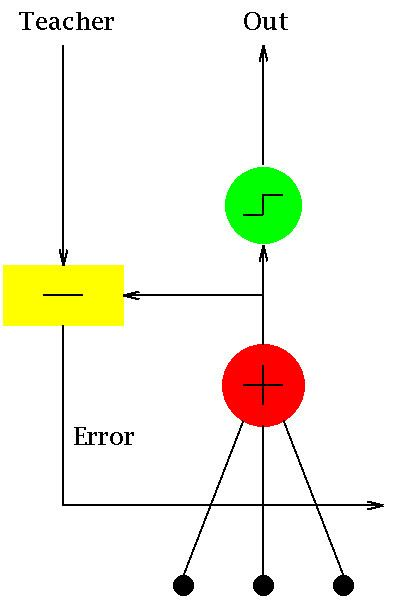Updated on
Covid-19ADALINE (Adaptive Lineardi Neuron or later Adaptive Linear Element) is an early single-layer artificial neural network and the name of the physical device that implemented this network. The network uses memistors. It was developed by Professor Bernard Widrow and his graduate student Ted Hoff at Stanford University in 1960. It is based on the McCulloch–Pitts neuron. It consists of a weight, a bias and a summation function.

## Contents

The difference between Adaline and the standard (McCulloch–Pitts) perceptron is that in the learning phase, the weights are adjusted according to the weighted sum of the inputs (the net). In the standard perceptron, the net is passed to the activation (transfer) function and the function's output is used for adjusting the weights.

There also exists an extension known as Madaline.

## Definition

Adaline is a single layer neural network with multiple nodes where each node accepts multiple inputs and generates one output. Given the following variables:as

• x is the input vector
• w is the weight vector
• n is the number of inputs
• θ some constant
• y is the output of the model
• then we find that the output is y = j = 1 n x j w j + θ . If we further assume that

• x n + 1 = 1
• w n + 1 = θ
• then the output further reduces to the dot product of x and w: y = x w

## Learning algorithm

Let us assume:

• η is the learning rate (some positive constant)
• y is the output of the model
• o is the target (desired) output
• then the weights are updated as follows w w + η ( o y ) x . The ADALINE converges to the least squares error which is E = ( o y ) 2 . This update rule is in fact the stochastic gradient descent update for linear regression.

## References

Similar Topics
The Witches (1966 film)